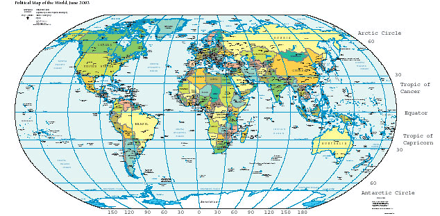## FANDOM

254,494 Pages

For the use of coordinates on Wikipedia pages see: Wikipedia:WikiProject Geographical coordinatesMap of Earth showing lines of latitude (horizontally) and longitude (vertically), Eckert VI projection; large version (pdf, 1.8MB)

A geographic coordinate system enables every location on the earth to be specified by the three coordinates of a spherical coordinate system aligned with the spin axis of the Earth.

## First and second dimensions: latitude and longitudeEditLatitude phi (φ) and Longitude lambda (λ)

Borrowing from theories of the ancient Babylonians, later expanded by the famous Greek thinker and geographer Ptolemy, a full circle is divided into 360 degrees (Borrowing from theories of the ancient Babylonians' angle) (360°).

• latitude (abbreviation: Lat.) is the angle at the centre of the coordinate system between any point on the earth's surface and the plane of the equator. Lines joining points of the same latitude are called parallels, and they trace concentric circles on the surface of the earth. Each pole is 90 degrees: the north pole 90° N; the south pole 90° S. The 0° parallel of latitude is designated the equator, an imaginary line that divides the globe into the Northern and Southern Hemispheres.
• longitude (abbreviation: Long.) is the angle east or west, at the centre of the coordinate system, between any point on the earth's surface and the plane of an arbitrary north-south line between the two geographical poles. Lines joining points of the same longitude are called meridians. All meridians are halves of great circles, and are not parallel: by definition they converge at the north and south poles. The line passing through the (former) Royal Observatory (near London in the UK) is the international zero-longitude reference line, the Prime Meridian. The antipodal meridian of Greenwich is both 180°W and 180°E.

By combining these two angles, the horizontal position of any location on Earth can be specified.

For example, Baltimore (in the USA) has a latitude of 39.3° North, and a longitude of 76.6° West (39.3° N 76.6° W). So, a vector drawn from the center of the earth to a point 39.3° north of the equator and 76.6° west of Greenwich will pass through Baltimore.

This latitude/longitude "webbing" is known as the common graticule. There is also a complementary transverse graticule (meaning the graticule is shifted 90°, so that the poles are on the horizontal equator), upon which all spherical trigonometry is ultimately based.

Traditionally, degrees have been divided into minutes (1/60th of a degree, designated by ′ or "m") and seconds (1/60th of a minute, designated by ″ or "s"). There are several formats for degrees, all of them appearing in the same Lat-Long order:

• DMS Degree:Minute:Second (49°30'00"-123d30m00s)
• DM Degree:Minute (49°30.0'-123d30.0m)
• DD Decimal Degree (49.5000°-123.5000d), generally with 4 decimal numbers.

To convert from DM or DMS to DD, decimal degrees = whole number of degrees, plus minutes divided by 60, plus seconds divided by 3600. DMS is the most common format, and is standard on all charts and maps, as well as global positioning systems and geographic information systems.

On a spherical surface at sea level, one latitudinal second measures 30.82 metres and one latitudinal minute 1849 metres. Parallels are each 110.9 kilometres away. The circles of longitude, the meridians, meet at the geographical poles, with the west-east width of a second being dependent on the latitude. On a spherical surface at sea level, one longitudinal second measures 30.92 metres on the equator, 26.76 metres on the 30th parallel, 19.22 metres in Greenwich (51° 28' 38" N) and 15.42 metres on the 60th parallel.

The width of one longitudinal degree on latitude $\scriptstyle{\phi}\,\!$ can be calculated by this formula (to get the width per minute and second, divide by 60 and 3600, respectively):

$\frac{\pi}{180^{\circ}}\cos(\phi)M_r,\,\!$

where Earth's average meridional radius $\scriptstyle{M_r}\,\!$ approximately equals 6,367,449 m. Due to the average radius value used, this formula is of course not precise due to Earth's flattening. You can get real width of a longitudinal degree on latitude $\scriptstyle{\phi}\,\!$ by:

$\frac{\pi}{180^{\circ}}\cos(\phi)\sqrt{\frac{a^4\cos(\phi)^2+b^4\sin(\phi)^2}{(a\cos(\phi))^2+(b\sin(\phi))^2}},\,\!$

where Earth's equatorial and polar radii, $\scriptstyle{a,b}\,\!$ equal 6,378,137 m, 6,356,752.3 m, respectively.

The equator is the fundamental plane of all geographic coordinate systems. All spherical coordinate systems define such a fundamental plane.

Latitude and longitude values can be based on several different geodetic systems or datums, the most common being the WGS 84 used by all GPS equipment. In other words, the same point on the earth’s surface can be described by different latitude and longitude values depending on the reference datum.

In popular GIS software, data projected in latitude/longitude is often specified via a 'Geographic Coordinate System'. For example, data in latitude/longitude with the datum as the North American Datum of 1983 is denoted by 'GCS_North_American_1983'.

## Third dimension: altitude, height, depthEdit

To completely specify a location on, in, or above the earth, one has to also specify the elevation, defined as the vertical position of the location relative to the centre of the reference system or some definition of the earth's surface. This is expressed in terms of the vertical distance to the earth below, but, because of the ambiguity of "surface" and "vertical", is more commonly expressed relative to a more precisely defined datum such as mean sea level (more precisely named geoid, a surface of constant gravity potential). The distance to the earth's center can be used both for very deep positions and for positions in space.

Other terms used with respect to the distance of a point from the earth's surface or some other datum are altitude, height, and depth.

## Geostationary coordinatesEdit

Geostationary satellites (e.g., television satellites ) are over the equator. So, their position related to Earth is expressed in longitude degrees. Their latitude does not change, and is always zero over the equator.